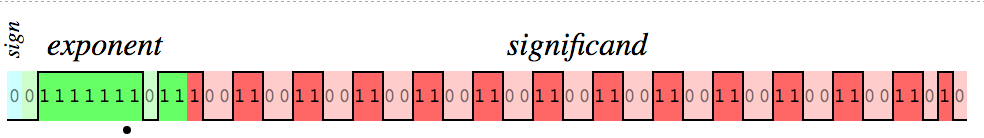# 10. The problem with simple math on computers¶

Have you ever considered how computers store numbers? Can you explain why this happens?

:

a = 0.1 + 0.1 + 0.1 + 0.1 + 0.1 + 0.1 + 0.1 + 0.1 + 0.1 + 0.1
a

:

0.9999999999999999

:

a == 1.

:

False

:

b = 0.125 + 0.125 + 0.125 + 0.125 + 0.125 + 0.125 + 0.125 + 0.125
b

:

1.0

:

b == 1.

:

True


## 10.1. Computers use base 2 instead of base 10¶

You’ve heard that computers are all about ones and zeros, right? What does this mean?

When I write a “normal” number like 123, what I mean is $$1\times10^2 + 2\times 10^1 + 3 \times 10^0$$. This idea is called base 10 or decimal representation. Computers use binary or base 2 representation. This means you would write $$101_2 = 5_10$$, with the subscript representing the base. The math would work out as $$1\times 2^2 + 0\times2^1 + 1\times 2^0$$, just like in the 123 example.

This representation is exact for integers, but we run into problems when we use fractions. For instance, we all know that $$1/3$$ doesn’t have a finite representation in decimals, since $$1/3 = 0.\overline{33} = 3\times 10^{-1} + 3\times10^{-2}+\cdots$$ forever. Notice that in base 3, 1/3 works out fine as $$0.1_3$$ since $$1/3 = 1\times3^{-1}$$ exactly. So here’s the problem with writing $$0.1$$ in binary:

This visualisation shows how IEEE floats are represented and indicates the repeating structure of the representation of 0.1.We can see that the binary representation is not finite, so the computer treats 1/10 more like a number like 1/7 (which we all know has an infinite decimal representation).

There is a great deal more information on this issue at these pages:

## 10.2. Solutions¶

### 10.2.1. Built-in to Python¶

The solution that Python supplies in the standard library is the decimal module:

:

import decimal

:

a = decimal.Decimal('0.1')

:

a = 1/decimal.Decimal(10)

:

sum(a for i in range(10))

:

Decimal('1.0')

:

sum(0.1 for i in range(10))

:

0.9999999999999999


### 10.2.2. Sympy¶

Sympy also has a solution in the form of a Rational object

:

import sympy
sympy.init_printing()

:

b = sympy.Rational('0.1')

:

b

:

$$\frac{1}{10}$$

We can also use sympy.nsimplify.

:

b = 1
c = 10

:

a = b/c

:

type(a)

:

float

:

sympy.nsimplify(a)

:

$$\frac{1}{10}$$

## 10.3. Why isn’t math always done in base 10?¶

The extra precision comes at a cost.

:

%%timeit
a = 0.1
s = 0
for i in range(100000):
s += a

5 ms ± 321 µs per loop (mean ± std. dev. of 7 runs, 100 loops each)

:

%%timeit
a = decimal.Decimal('0.1')
s = decimal.Decimal(0)
for i in range(100000):
s += a

10.8 ms ± 246 µs per loop (mean ± std. dev. of 7 runs, 100 loops each)

:

%%timeit
a = sympy.Rational(1, 10)
s = 0
for i in range(100000):
s += a

2.03 s ± 166 ms per loop (mean ± std. dev. of 7 runs, 1 loop each)


Using sympy rationals is about a thousand times slower than using built-in Python floats.

## 10.4. Forcing rounding of exact representations¶

If an equation results in an “Exact” answer which isn’t “useful”, like $$\sqrt{3}x$$, we can approximate that using sympy.N

:

x = sympy.Symbol('x')

:

expr = sympy.sqrt(3)*x
expr

:

$$\sqrt{3} x$$
:

sympy.simplify(expr**2)

:

$$3 x^{2}$$
:

sympy.simplify(sympy.N(expr, 3)**2)

:

$$3.0 x^{2}$$
[ ]: